## A collection of Python snippets for 3D

If you’re interested in taking the first step into Python for 3D software,
Or simply would like to browse some script examples, your welcome to visit my Gist page,
It contains a useful library of Python code example for Blender, Maya, 3ds max and Unreal engine:
https://gist.github.com/CGLion

## Writing a basic OSL color shader

The following is an introduction to basic OSL shader syntax using a simple color blending shader example. a more general explanation of the subject can be read here.

Notes:
> It’s highly recommended to get acquainted with basic C language syntax, since it’s the basis for common shading languages like OSL, HLSL and GLSL.
> More detailed information about writing OSL shaders can be found in the osl-languagespec PDF document from ImageWorks’s OSL GitHub.

This example shader blends 2 color sources according to the surface viewing angle (aka “facing ratio” or “incident angle” or “Perpendicular-Parallel”). the user can choose a facing (“front”) color or texture, a side color or texture, and the shader’s output bell be a mix of the these 2 inputs that depends on the angle the surface is viewed at.

``` #include "stdosl.h"

[[ string help = "Blend colors by view angle" ]]
(
color facing_color = color(0, 0, 0)
[[ string help = "The color (or texture) that will appear at perpendicular view angle" ]],
color side_color = color(1, 1, 1)
[[ string help = "The color (or texture) that will appear at grazing view angle" ]],
float base_blend = 0.0
[[ string help = "The percent of side_color that is mixed with facing_color at perpendicular view angle",
float min = 0.0, float max = 1.0]],
float curve_exponent = 1.0
[[ string help = "A power exponent value by which the blend value is raised to control the blend curve",
float min = 0.001, float max = 10.0]],
output color color_out = color(1, 1, 1))
{
// calculate the linear facing ratio:
float facing_ratio = acos(abs(dot(-I,N))) / M_PI_2;
// calculate the curve facing ratio:
float final_blend_ratio = pow(facing_ratio , curve_exponent);
// blend the facing color:
color final_facing_color = (facing_color * (1 - base_blend)) + (side_color * base_blend);
// blend and output the final color:
color_out = ((final_facing_color * (1 - final_blend_ratio)) + (side_color * final_blend_ratio));
}```

The first statment:

`#include "stdosl.h"`

The #include statement is a standard C compiler directive to link the OSL source code library code file stdosl.h to the shader’s code, so that the OSL data types and functions in the code will be recognized.
* Some systems compile the code successfully without this statement. I’m not sure if their compiler links stdosl.h automatically or not.

The double-square bracketed statements provide both help annotations and value range limits for the shader parameters:

`[[ string help = "The percent of side_color that is mixed with facing_color at perpendicular view angle", float min = 0.0, float max = 1.0]]`

Note that these statements are appended to single parameters in the shader right before the comma character that ends the parameter statement.
* Not all shading systems that supprt OSL also implement the annotations in the shader’s interface generated by the host software (the shader will work, but it’s parameters wont be described and limited to the defined value range).

Removing the #include statement, annotations and comments,
We can see that the OSL shader structure is very similar to a C function:

```shader cglColorAngleBlend
(
color facing_color = color(0, 0, 0),
color side_color = color(1, 1, 1),
float base_blend = 0.0,
float curve_exponent = 1.0,
output color color_out = color(1, 1, 1)
)
{
float facing_ratio = acos(abs(dot(-I,N))) / M_PI_2;
float final_blend_ratio = pow(facing_ratio , curve_exponent);
color final_facing_color = (facing_color * (1 - base_blend)) + (side_color * base_blend);
color_out = ((final_facing_color * (1 - final_blend_ratio)) + (side_color * final_blend_ratio));
}```

First the data type, in this case shader, followed by the shader identifier, in this case “cglColorAngleBlend”:

`shader cglColorAngleBlend`

After the shader’s type and identifier, a list of parameters is defined within parentheses, separated by comma’s. these parameters define the shader’s input’s, outputs, and default values. Output parameters are preceded by the output modifier.

(
<parameter type> <parameter identifier> = <parameter default value>,
<parameter type> <parameter identifier> = <parameter default value>,
output <parameter type> <parameter identifier> = <parameter default value>
)

```(
color facing_color = color(0, 0, 0),
color side_color = color(1, 1, 1),
float base_blend = 0.0,
float curve_exponent = 1.0,
output color color_out = color(1, 1, 1)
)```

In this case the shader has 4 user input parameters, and 1 output parameter.
2 color type parameters, “facing_color” and “side_color” for the facing and side color that will be blended together, a float* parameter “base_blend” that specifies how much of the side color will be mixed with the facing color regardless of view angle, and a second float parameter “curve_exponent” specifying a power exponent by which the blend value will be raised to create a non-linear blend curve.
The output parameter “color_out” is a color that will calculated by the shader.
* Note that even though the the output parameter will be calculated by the shader, it is required to define a default value for it for the shader to compile.

After the shader parameters, enclosed within curly braces is the actual body of the shader code, containing the instructions, each ending by a semicolon ; character:

{
<instruction>;
<instruction>;
…..
}

```{
float facing_ratio = acos(abs(dot(-I,N))) / M_PI_2;
float final_blend_ratio = pow(facing_ratio , curve_exponent);
color final_facing_color = (facing_color * (1 - base_blend)) + (side_color * base_blend);
color_out = ((final_facing_color * (1 - final_blend_ratio)) + (side_color * final_blend_ratio));
}```

I the case of our shader the first code instruction is to define a new float internal variable named “facing_ratio”, calculate the surface/view angle and assign the resulting value to it:

`float facing_ratio = acos(abs(dot(-I,N))) / M_PI_2;`

The expression:

acos( abs( dot( -I, N ) ) )  / M_PI_2

calculates incident angle, i.e. angle between 2 vectors, originating at the surface shading point, one pointing towards the origin of the incoming ray, and the other the surface normal, as a factor of 0 to 1 representing 0 – 90 degrees.
These 2 vector are easily obtained through the built in OSL global variables N and I. N is the surface normal at the shading point, and I is the incoming ray vector pointing to the shading point which is inverted in this case to point backwards by  typing a minus before it: -I.
The incident angle is calculated in radians as the arc-cosine of the dot-product of N and -I and then divided by half a π to convert it to a linear factor of 0 to 1 representing 0 to 90 degrees in radians, M_PI_2 being a convenient half π constant.
* M_PI being a full π, M_2PI being 2π representing 180 degrees in radians and 360 degrees respectively (OSL provides there are more constants in this series).

The second instruction raises the facing ratio that was calculated in the previous instruction by a power value provided by the curve_exponent input parameter, to create a non linear angle/color blend in values other than 1.0.
The resulting modified blend value is stored in a new internal variable final_blend_ratio:

`float final_blend_ratio = pow(facing_ratio , curve_exponent);`

Note:
We could avoid setting a new variable by modifying the value of  the facing_ratio variable, and we could also combine the these 2 instruction into 1 bigger expression like this:

pow( acos( abs( dot( -I, N ) ) ) / M_PI_2 , curve_exponent )

But I decided to keep it separated into 2 variable and 2 instructions for clarity.
* try modifying the code as an exercise

The third instruction modifies the input color facing_color by premixing it with the input side_color according to the percent give by the input parameter base_blend and assigns the resulting color to a new internal variable named final_facing_color:

`color final_facing_color = (facing_color * (1 - base_blend)) + (side_color * base_blend);`

The expression:

( facing_color * ( 1 – base_blend ) ) + ( side_color * base_blend )

Calculates a linear combination** (linear interpolation) between the 2 input colors using the base_blend as a 0 – 1 factor between them.
* Note that OSL allows to define arithmatic operations freely between colors and floats.

The forth and final instruction creates the final mix between the modified facing color stored in final_facing_color variable and the side color given by the input color parameter side_color, by again, calculating a linear combination between the 2 colors, this time using final_blend_ratio variable value we calculated previously as the combination factor, and very importantly, finally, assigning the mixed result to the shader output parameter color_out so it will be the final output of the shader:

`color_out = ((final_facing_color * (1 - final_blend_ratio)) + (side_color * final_blend_ratio));`

This screen capture shows this shader at work in Blender and Cycles, connected to a Principled BSDF shader as it’s base color source:Thats it! 🙂

Clarifications:

* A “float” data type is simply the the computer-science geeky way of saying “accurate non-integer number”. when we have to store numbers that can describe geometry and color, we need a data type that isn’t limited to integers so for that purpose we use float values. there’s actually a lot more to the float formal definition in computer science, but for our purpose here this will suffice.

** A Linear Combination, or Linear interpolation (lerp) is one of the most useful numerical operations in 3D geometry and color processing (vector math):
A * ( 1 – t ) +  B * t
A and B being your source and target locations or colors or any other value you need to interpolate and t being the blending factor from 0 – 1.

Related posts:

## Using OSL Shaders in Blender & Cycles

Software:
Blender 2.82 | Cycles

The Cycles render engine in Blender has a very convenient OSL Shader development and usage workflow.
Shaders can be both loaded from external files or written and compiled directly inside Blender.

Before you begin:
Make sure your Blender scene is set to use the Cycles render engine, in CPU rendering mode, and also check the option Open Shading Language:To write an OSL shader in Blender:Create a Script node: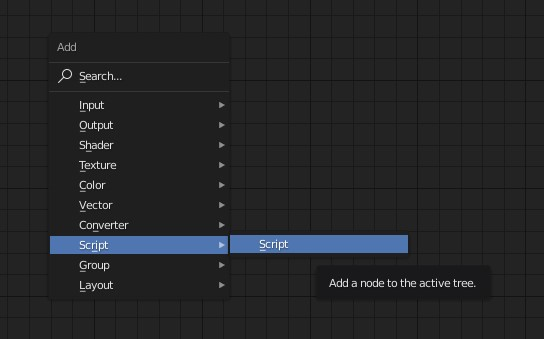3. Set the Script node‘s mode to Internal,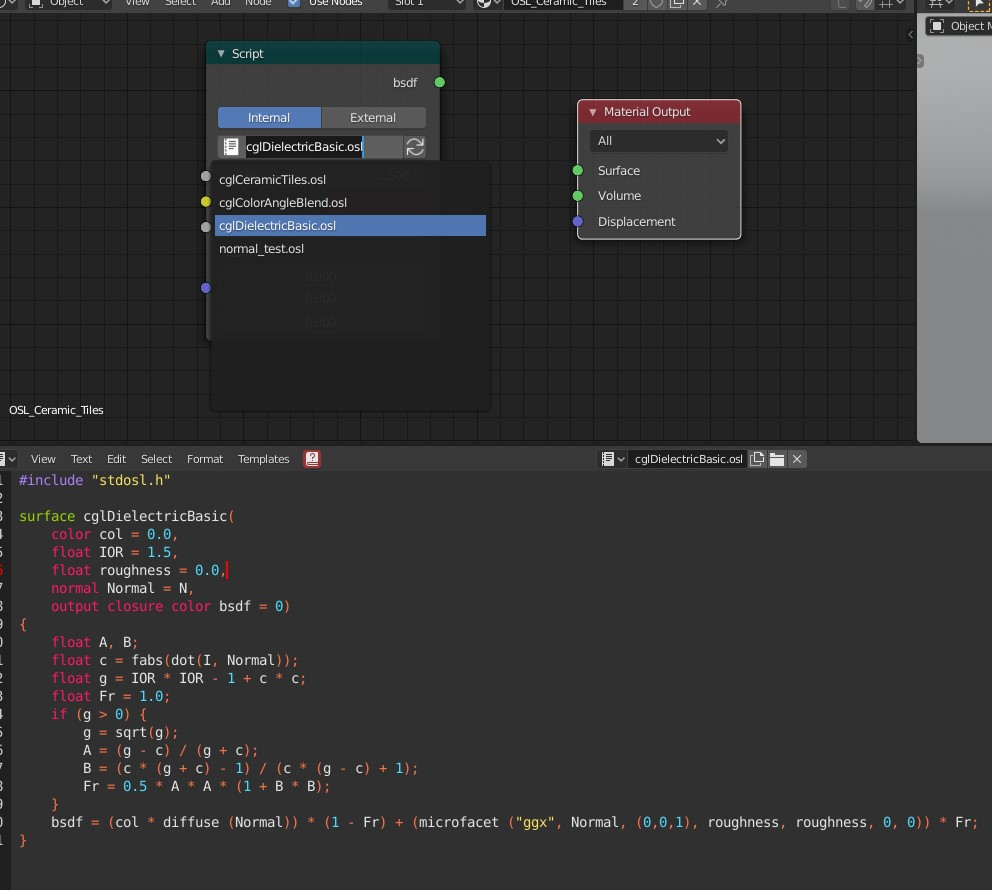4. If the shader compiles successfully, the Script node will display its input and output parameters, and you can connect it’s output to an appropriate input in your shading graph.
* If your shader is a material (color closure) connect it directly to the Material Output node’s Surface input, is it’s a volume to the Volume input, or if its a texture to other material inputs as needed.5. If the shader code contains errors, it will fail to compile, and you’l be able to read the error messages in Blender‘s System Console window: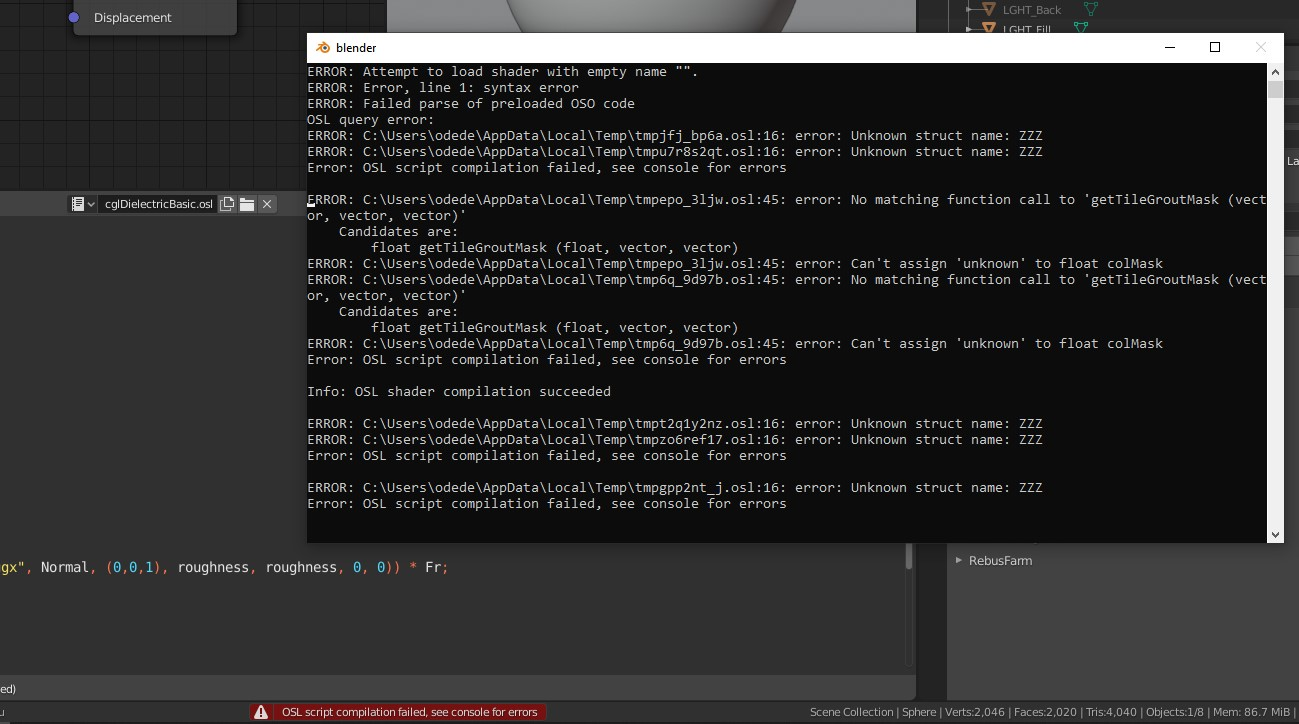6. After fixing errors or updating the shader’s code, press the Script Noe Update button on the Script node to re-compile the shader: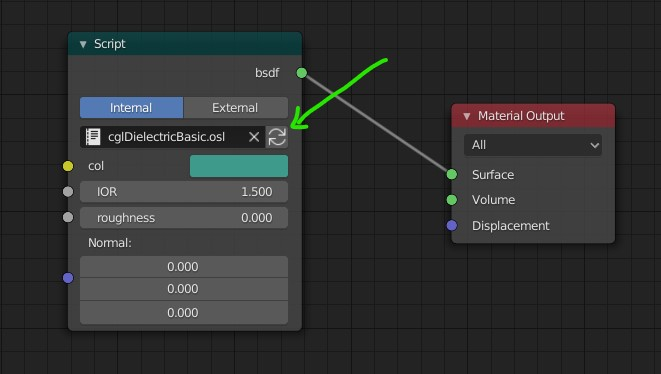Exactly the same workflow described in the previous section, except setting the Script node‘s mode to External and either typing a path to the shader file in the Script node or pressing the little folder button to locate it using the file browser: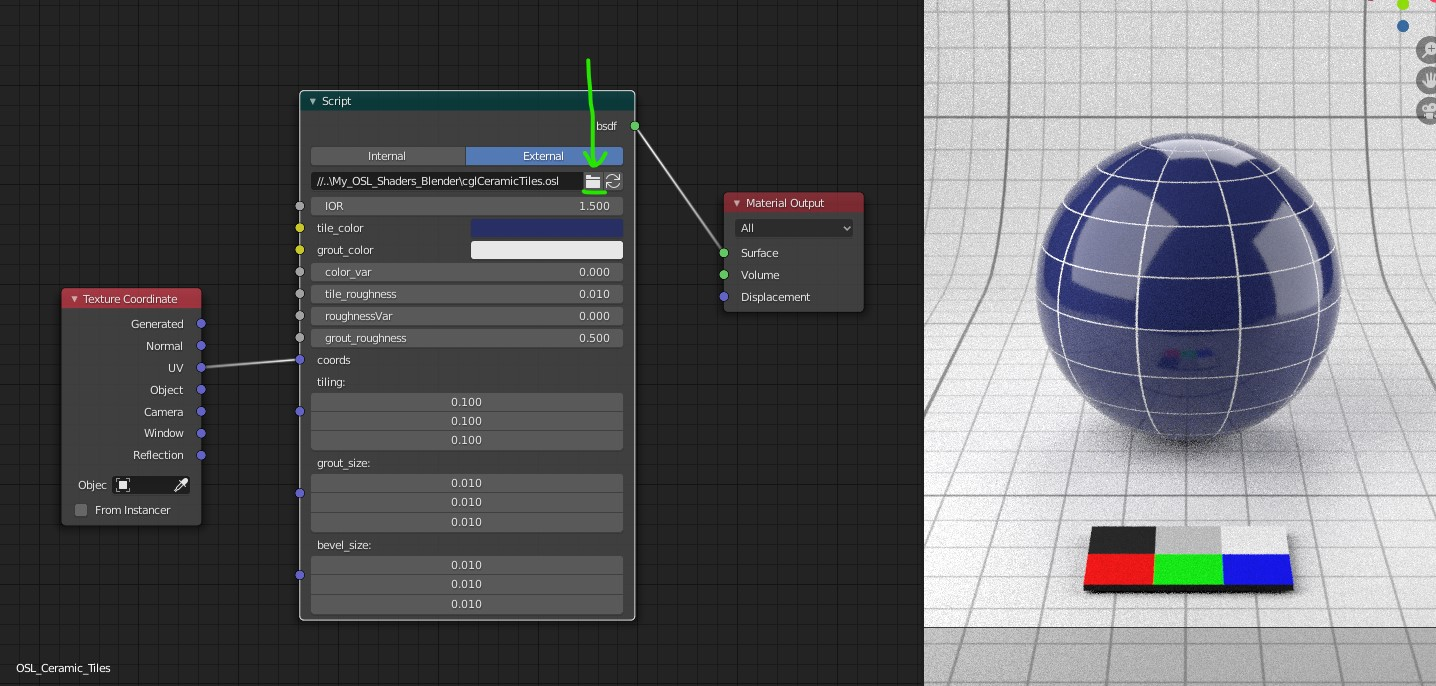Related:

Software:
3ds max 2020

An example of loading and displaying an image file using Python for 3ds max:
* The EXR image file is located in in the same directory with the 3ds max file in this case.
```from MaxPlus import BitmapManager
image_file_path = r'BG_park_A.exr'
bmp_storage = MaxPlus.Factory.CreateStorage(17)
bmp_info = bmp_storage.GetBitmapInfo()
bmp_info.SetName(image_file_path)
bmp.Display()```

Script explanation line by line:
1. Import the BitmapManager class needed to load image files.
2. Set a variable containing the path to the image file
3. Call the MaxPlus.Factory class’s CreateStorage method to initiate a BitmapStorage object.
This is embarrassing IMO..
And it may very well be that I simply didn’t find the correct way it should be done..
I couldn’t find any other way to independently initiate the BitmapInfo object needed for loading the image, other than Initiating a BitmapStorage object and getting referece to its BitmapInfo object. (the BitmapInfo class has no constructor..)
* If you know a better method to do this I’ll be very grateful if you take the time to comment.
Note:
that the 17 integer argument we supply sets the storage to be compatible with:
32-bit floating-point color depth format (without an alpha channel).
See list of other color format options in this example here:
https://help.autodesk.com/view/3DSMAX/2020/ENU/?guid=__developer_using_maxplus_creating_a_bitmap_html
* They wrote a class containing convenient named constants of the integer arguments (see example code below).
* In this example of creating the BitmapStorage just as a way to generate a BitmapInfo object the actual format you’l supply doesn’t matter, but you can’t use a format that can’t be written to like 8 for example (see list below)
4. Get a reference to the BitmapInfo object contained in the BitmapStorage object.
5. Setting the name property (full file path) of the BitmapInfo object.
7. Displaying the image in 3ds max‘s image viewer window.

Example code for a BitmapStorage format constants container class:

* This example’s source is in the 3ds max help Python examples:
https://help.autodesk.com/view/3DSMAX/2020/ENU/?guid=__developer_using_maxplus_creating_a_bitmap_html
```class BitmapTypes(object):
BMM_NO_TYPE = 0 # Not allocated yet
BMM_LINE_ART = 1 # 1-bit monochrome image
BMM_PALETTED = 2 # 8-bit paletted image. Each pixel value is an index into the color table.
BMM_GRAY_8 = 3 # 8-bit grayscale bitmap.
BMM_GRAY_16 = 4 # 16-bit grayscale bitmap.
BMM_TRUE_16 = 5 # 16-bit true color image.
BMM_TRUE_32 = 6 # 32-bit color: 8 bits each for Red, Green, Blue, and Alpha.
BMM_TRUE_64 = 7 # 64-bit color: 16 bits each for Red, Green, Blue, and Alpha.
BMM_TRUE_24 = 8 # 24-bit color: 8 bits each for Red, Green, and Blue. Cannot be written to.
BMM_TRUE_48 = 9 # 48-bit color: 16 bits each for Red, Green, and Blue. Cannot be written to.
BMM_YUV_422 = 10 # This is the YUV format - CCIR 601. Cannot be written to.
BMM_BMP_4 = 11 # Windows BMP 16-bit color bitmap. Cannot be written to.
BMM_PAD_24 = 12 # Padded 24-bit (in a 32 bit register). Cannot be written to.
BMM_LOGLUV_32 = 13 BMM_LOGLUV_24 = 14
BMM_LOGLUV_24A = 15 BMM_REALPIX_32 = 16 # The 'Real Pixel' format.
BMM_FLOAT_RGBA_32 = 17 # 32-bit floating-point per component (non-compressed),
RGB with or without alpha
BMM_FLOAT_GRAY_32 = 18 # 32-bit floating-point (non-compressed), monochrome/grayscale
BMM_FLOAT_RGB_32 = 19
BMM_FLOAT_A_32 = 20```

Reading pixel values from the image:
```bmp_storage = bmp.GetStorage()
hdr_pixel = bmp_storage.GetHDRPixel(3000,200)
print(hdr_pixel)```
1. Get reference to the Bitmap‘s BitmapStorage object.
* in this case, writing over the BitmapStorage object we created earlier just to get the BitmapInfo object..

Note:

When copying and pasting a script from this example, the indentation may not be pasted correctly.

Related:

## Blender – Basic time-dependent animation driver examples

Software:
Blender 2.82

To setup a time-dependent Driver in Blender, simply use the built-in frame variable.
In this example the expression:

`sin(frame)`

Set as a Z axis location driver for the cube causes it to oscillate up and down:Changing the expression to:

`sin( frame * 0.1 ) * 2`

Causes the motion to be twice as high and 10X slower: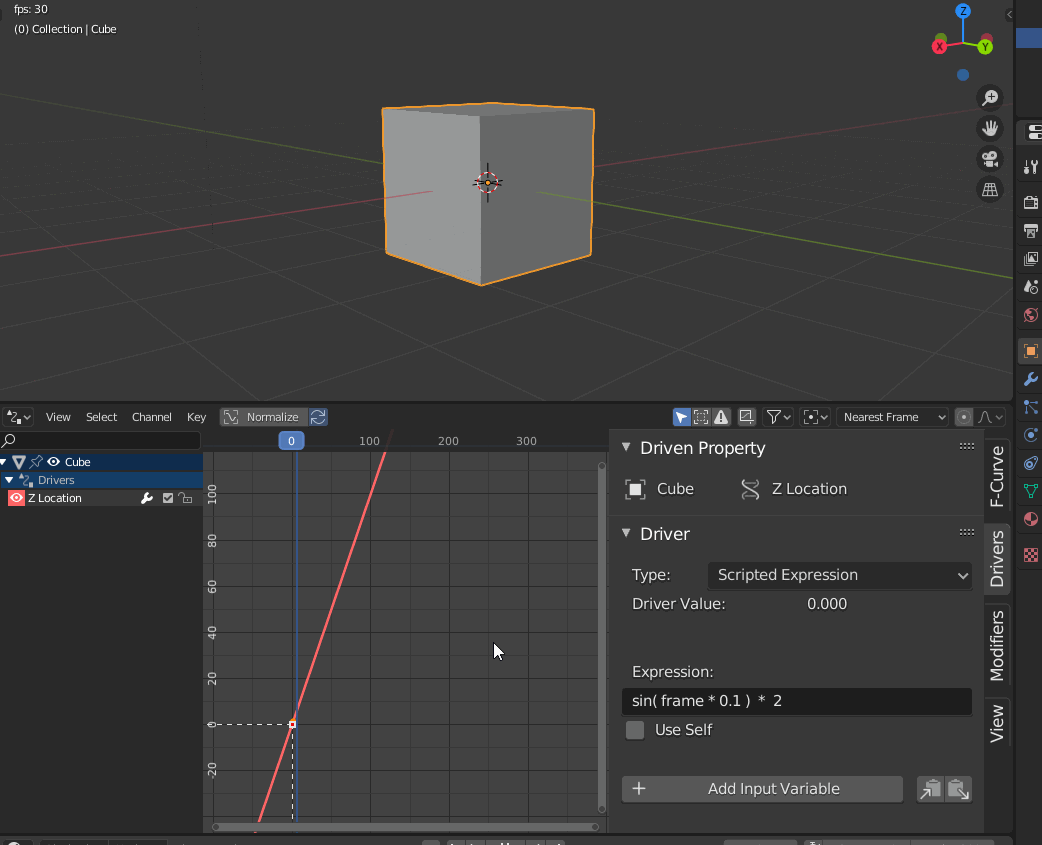In this example, the expression:

`( pow( -1 ,  floor( frame / 30 ) )  *  0.5 ) + 0.5`

Set to the cube’s Emission shader’s Strength attribute causes it to alternate between values of 0 and 1 every second (30 frames in this case):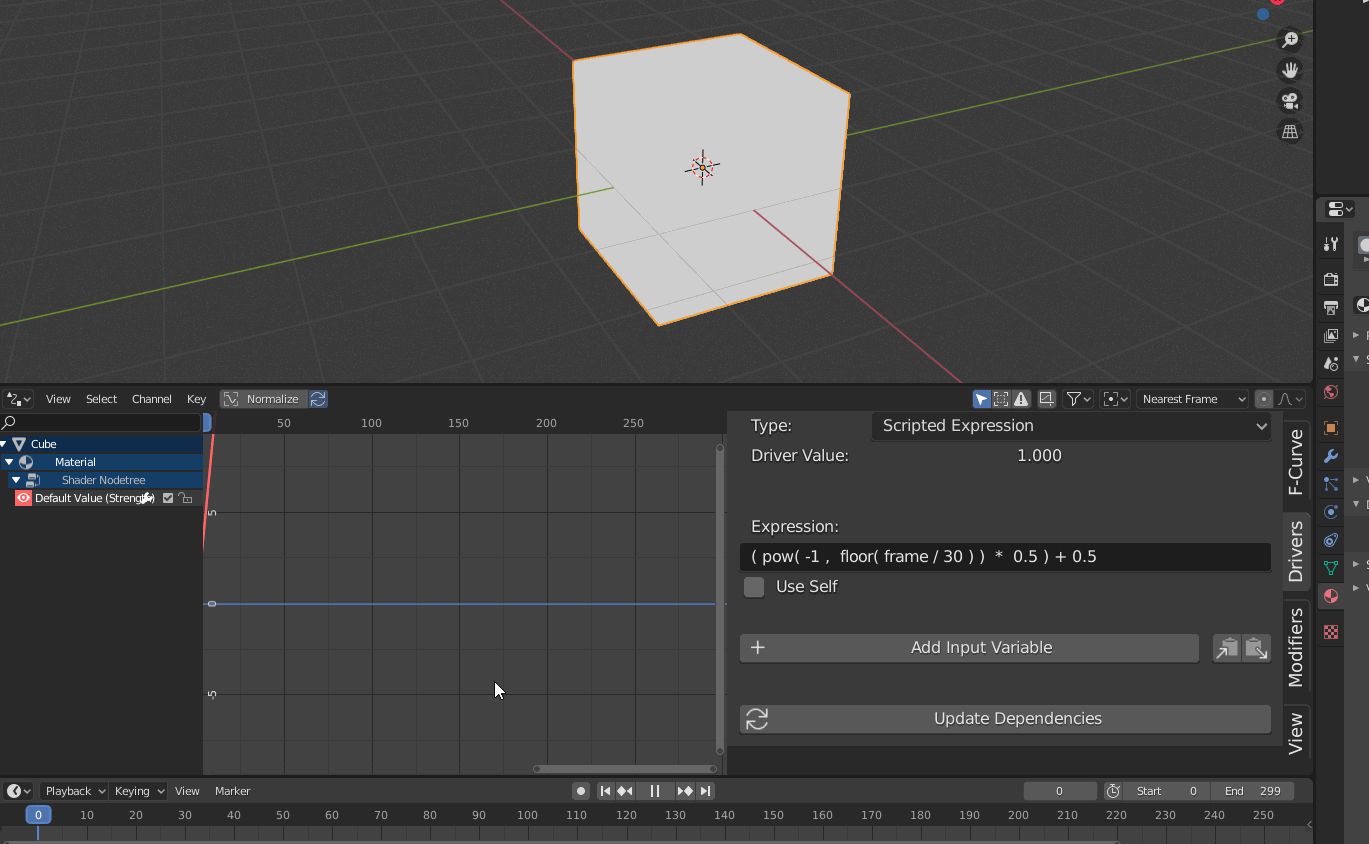## Python for Blender – Batch renaming objects

Software:
Blender 2.8 | Python 3.7

Some useful short Python snippets for batch re-naming objects in Blender:

1. Remove last 4 characters from selected object names:
```import bpy
objects = bpy.context.selected_objects
for o in objects:
o.name = o.name[:-4]```2. Rename all selected objects to a set base name followed by a 3 digit numeric suffix:
```import bpy
objects = bpy.context.selected_objects
for (i,o) in enumerate(objects):
o.name = "some_base_name_{:03d}".format(i)```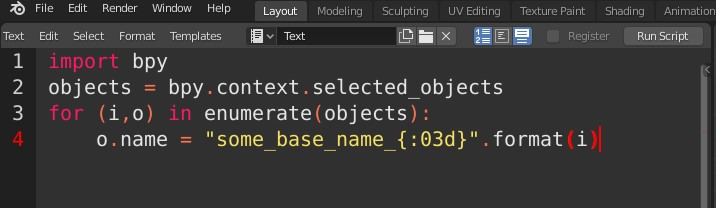3. Prefix all selected objects name with their Blender data type like renaming “SomeModel” to “MESH_SomeModel” or for example:
```import bpy
objects = bpy.context.selected_objects
for (i,o) in enumerate(objects):
o.name = "{}_{}".format(o.type,o.name)```* note that when copying and pasting a script from this example, the indentation may not be pasted correctly.

Note:
Blender 2.8 has a robust batch renaming utility that is invoked by pressing Ctrl + F2 so we don’t have to write scripts to do batch renaming of objects:## Python for 3ds max – Select objects of type

Software:
3ds max 2020

Continuing this example,
If you need to select objects (nodes) of a certain type i.e. lights, cameras etc.
You can use the INode class’s GetObject() method to get a reference to the node’s object, or “modified object” in 3ds max terms, and use the object’s GetSuperClassID() method to get the integer id representing the object’s superclass.

In addition, the MaxPlus SuperClassIds class contains convenient constants that can be used to avoid having to check and remember the superclasses numeric ids.
See reference here:
https://help.autodesk.com/view/3DSMAX/2017/ENU/?guid=__py_ref_class_max_plus_1_1_super_class_ids_html

An example of a script that selects all light objects in the scene:

```from MaxPlus import SuperClassIds
from MaxPlus import SelectionManager

def scene_objects():
def list_children(node):
list = []
for c in node.Children:
list.append(c)
list = list + list_children(c)
return list
return list_children(MaxPlus.Core.GetRootNode())

for o in scene_objects():
obj = o.GetObject()
if obj.GetSuperClassID() == SuperClassIds.Light:
SelectionManager.SelectNode(o, False)```

* note that when copying and pasting a script from this example, the indentation may not be pasted correctly.

## PyCharm – Enabling Scroll Zoom

Software:
PyCharm Community 2019.1

Unlike many popular text/programming editors and IDE’s, PyCharm doesn’t by default let you change the font size by pressing Ctrl + Scrolling the mouse wheel.

To enable scroll-zoom in PyCharm:

1. Go to File > Settings to open the Settings dialog
2. In the Settings dialog, navigate to Editor > General
3. Check the Change font size (Zoom) with Ctrl + Mouse Wheel option.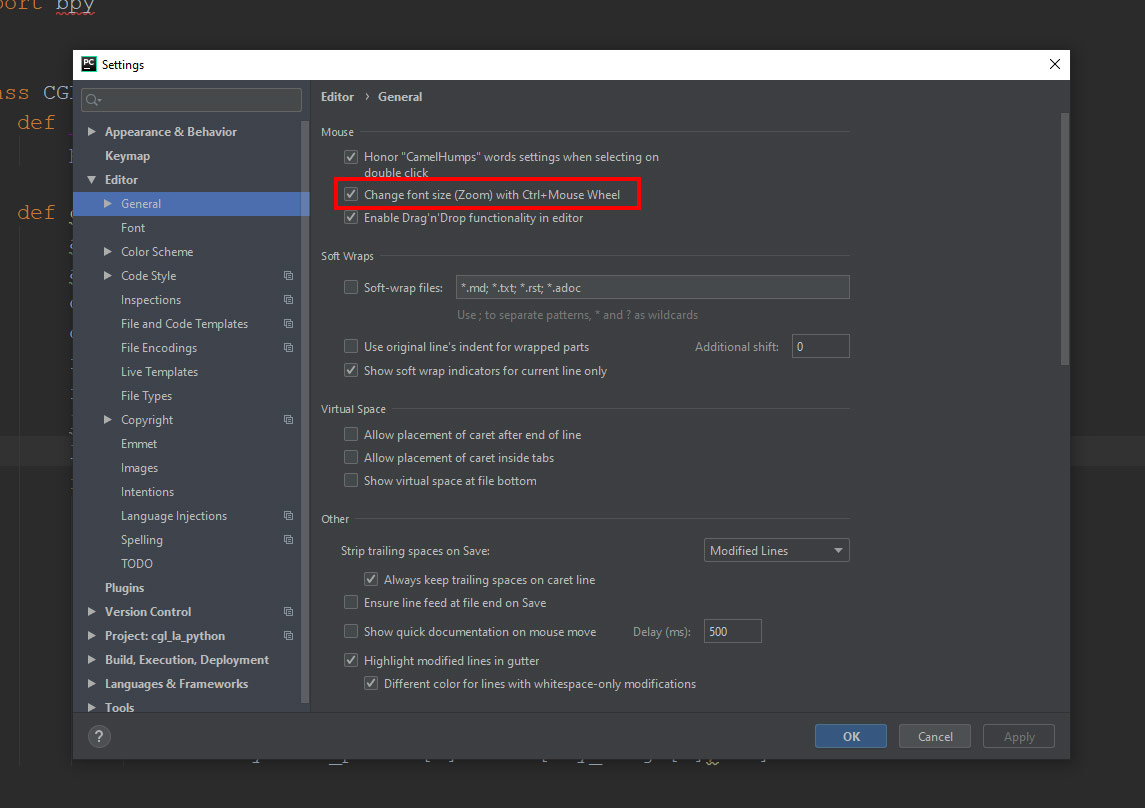## Python for 3ds max – Mesh manipulation

Software:
3ds max 2019

An example of creating a mesh ripple deformation using Python for 3ds max: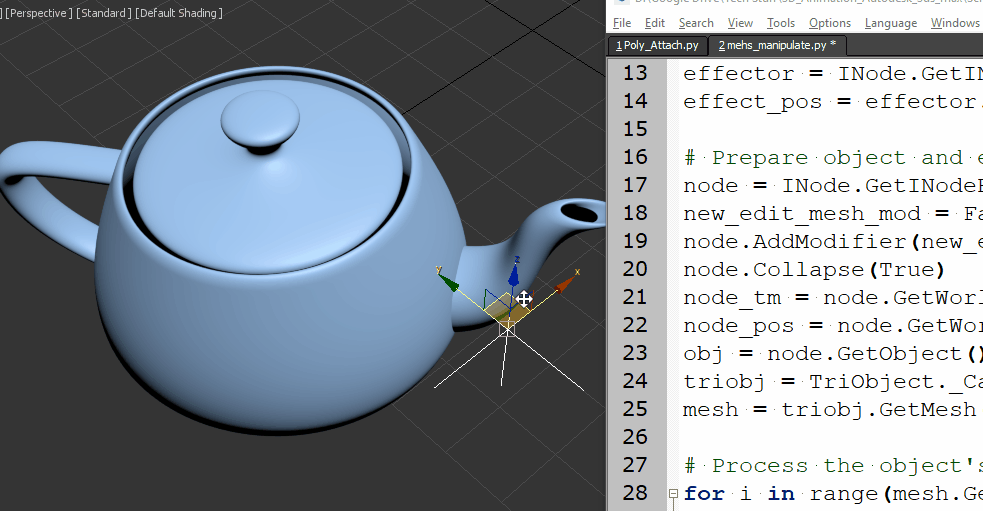Script steps:

1. Define the effect intensity and a helper point object that will serve as the effect center.
2. Collapse the object to an Editable-Mesh so its vertices will be accessible by Python.
Note that the Node‘s Object has to be cast as a TriObject, to access the object’s Mesh data.
3.  Loop through the Mesh’s vertices, get their world position, and set a new Z position as a sine function of the distance from the effect center.
```import math
from MaxPlus import Factory
from MaxPlus import ClassIds
from MaxPlus import INode
from MaxPlus import TriObject
from MaxPlus import Matrix3
from MaxPlus import Point3

# Intensity:
effecr_mult = 1.0

# Effect center:
effector = INode.GetINodeByName('Point001')
effect_pos = effector.GetWorldPosition()

# Prepare object and eccess it's mesh data:
node = INode.GetINodeByName('Teapot001')
new_edit_mesh_mod = Factory.CreateObjectModifier(ClassIds.Edit_Mesh)
node.Collapse(True)
node_tm = node.GetWorldTM()
node_pos = node.GetWorldPosition()
obj = node.GetObject()
triobj = TriObject._CastFrom(obj)
mesh = triobj.GetMesh()

# Process the object's vertices:
for i in range(mesh.GetNumVertices()):
# Get vertex in world space
vert_pos = mesh.GetVertex(i)
vert_world_pos = node_tm.VectorTransform(vert_pos)
vert_world_pos = vert_world_pos + node_pos
# Get vertex distance from effect center:
diff_vec = vert_world_pos - effect_pos
diff_vec.Z = 0
dist = diff_vec.GetLength()
# Set new vertex position:
mesh.SetVert(i,vert_pos.X,vert_pos.Y,vert_pos.Z + math.sin(dist)*effecr_mult)```

* note that when copying and pasting a script from this example, the indentation may not be pasted correctly.

Related Post:
Python for 3ds max – Animated Mesh

## UE4 – Python – Importing assets

Software:
Unreal Engine 4.20Importing assets into a project is done using the import_asset_tasks() function which is a member of the unreal.AssetTools class.
A reference to the AssetTools class is created by calling the get_asset_tools() function which is a member of the unreal.AssetToolHelpers class.
The import_asset_tasks() function requires a list of unreal.AssetImportTask objects as an argument, each unreal.AssetImportTask object in the supplied list represents the import action of a single asset, and contains properties needed for the import operation.
Asset import properties are set using the set_editor_property() function which is called through the AssetImportTask object.
Available asset import properties are listed here:

In the following example a specified texture file is imported into the project and stored in the Content(Game) > Textures folder.
* If the folder doesn’t exist it will be created.

```import unreal
AssetTools = unreal.AssetToolsHelpers.get_asset_tools()

The following example imports all the JPG files from folder: D:\ into the project, stores the new assets in Content(Game)\Textures folder and saves them:

```from os import listdir
from os.path import isfile, join
import unreal
dir = "D:\\"
files = [f for f in listdir(dir) if isfile(join(dir, f)) and f[-3:]=='jpg']
AssetTools = unreal.AssetToolsHelpers.get_asset_tools()